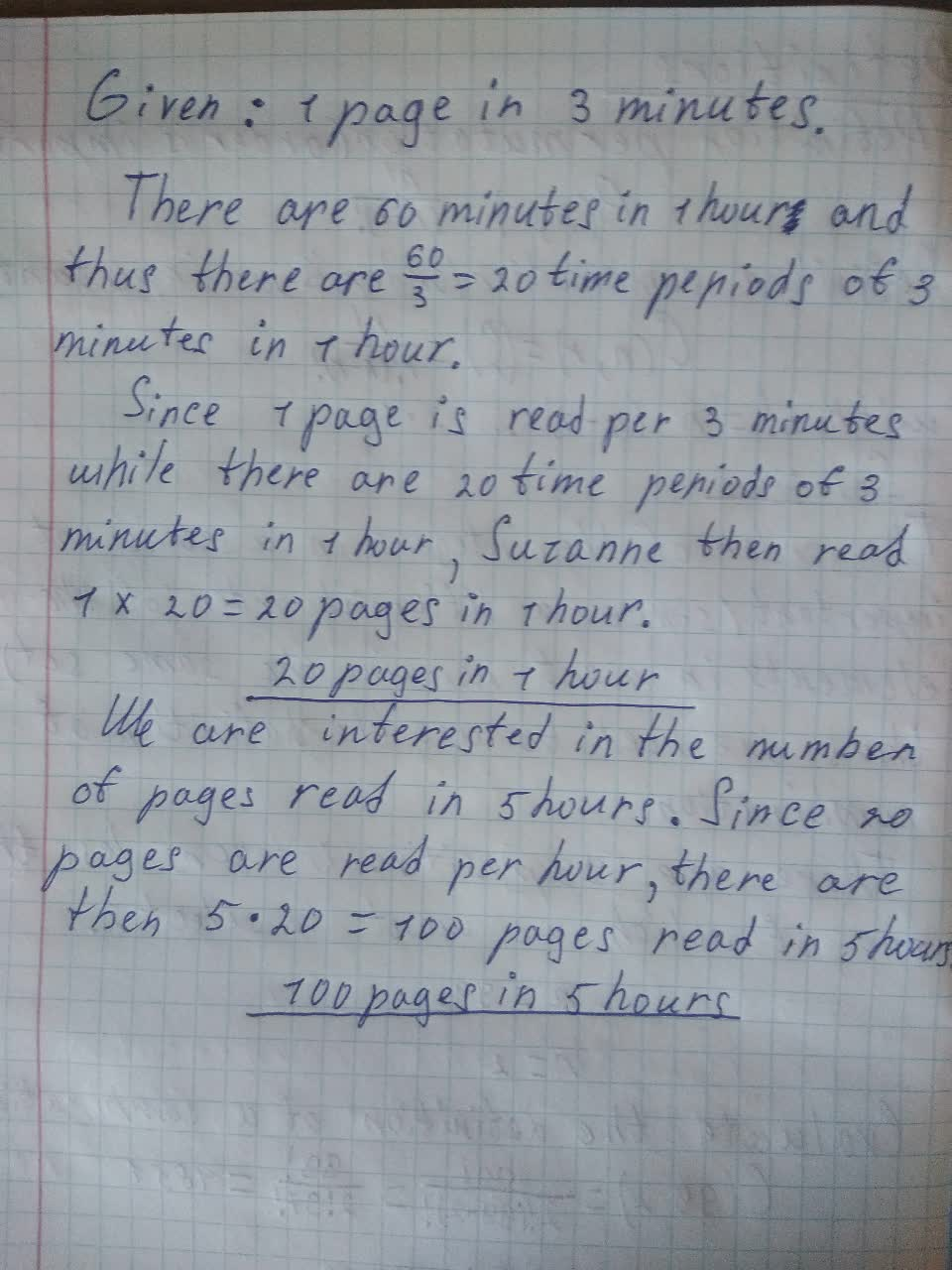# To calculate: Suzanne can read 1 page in 3 minutes. How many pages can she read in 5 hours?Brittney Lord 2021-08-02 Answered
Suzanne can read 1 page in 3 minutes. How many pages can she read in 5 hours?

• Questions are typically answered in as fast as 30 minutes

### Plainmath recommends

• Get a detailed answer even on the hardest topics.
• Ask an expert for a step-by-step guidance to learn to do it yourself.un4t5o4v###### Have a similar question?content_user

Given: 1 page in 3 minutes.

There are 60 minutes in 1 hour and thus there are $$\frac{60}{3}=20$$ time periods of 3 minutes in 1 hour.

Since 1 page is read per 3 minutes while there are 20 time periods of 3 minutes in 1 hour, Suzanne then read $$1\times20=20$$ pages in 1hour.

We are interested in the number of pages read in 5 hours. Since 20 pages are read per hour, there are then $$5\cdot20=100$$ pages read in 5 hours